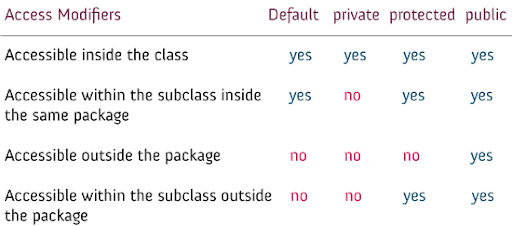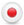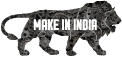Java Access Modifiers Lesson
Wednesday, October 15, 2008

# Java Access Modifiers Lesson

A modifiers assigns characteristics to the methods, data and variables. It specifies the availability of the method to other methods or classes. Variables and methods can be at any of the following access levels:

Default | Public | Private | Protected

public :

No doubt public variable any one can acess, class or methods are universally accessible.

Oxigen public propery...default :

Classes can be at default level. If you do not give any explicit modifier to a variable, class or method they will be automatically treated as Default.
It means that access is permitted from any method only in classes that are members of the same package as the target.

Eg:

class 9lesson
{
int i; (Default)
}

private :

private variables or methods can be accessed only from the methods of the class to which it belongs. A subclass also does not have access to the private variables.

Debit card having security pin numberprotected :

a protected variable or method is accessible from any class of the same package or from any subclass is in a different package.

This is like father and child relation.Download Source

The first class is SubclassInSamePackage.java which is present in pckage1 package. This java file contains the Base class and a subclass within the enclosing class that belongs to the same class as shown below.

package pckage1;

class BaseClass {

public int x = 10;
private int y = 10;
protected int z = 10;
int a = 10; //Implicit Default Access Modifier
public int getX() {
return x;
}
public void setX(int x) {
this.x = x;
}
private int getY() {
return y;
}
private void setY(int y) {
this.y = y;
}
protected int getZ() {
return z;
}
protected void setZ(int z) {
this.z = z;
}
int getA() {
return a;
}
void setA(int a) {
this.a = a;
}
}

public class SubclassInSamePackage extends BaseClass {

public static void main(String args[]) {
BaseClass rr = new BaseClass();
rr.z = 0;
SubclassInSamePackage subClassObj = new SubclassInSamePackage();
//Access Modifiers - Public
System.out.println("Value of x is : " + subClassObj.x);
subClassObj.setX(20);
System.out.println("Value of x is : " + subClassObj.x);
//Access Modifiers - Public
// If we remove the comments it would result in a compilaton
// error as the fields and methods being accessed are private
/* System.out.println("Value of y is : "+subClassObj.y);

subClassObj.setY(20);

System.out.println("Value of y is : "+subClassObj.y);*/
//Access Modifiers - Protected
System.out.println("Value of z is : " + subClassObj.z);
subClassObj.setZ(30);
System.out.println("Value of z is : " + subClassObj.z);
//Access Modifiers - Default
System.out.println("Value of x is : " + subClassObj.a);
subClassObj.setA(20);
System.out.println("Value of x is : " + subClassObj.a);
}
}

Output

Value of x is : 10
Value of x is : 20
Value of z is : 10
Value of z is : 30
Value of x is : 10
Value of x is : 20

The second class is SubClassInDifferentPackage.java which is present in a different package then the first one. This java class extends First class (SubclassInSamePackage.java).

import pckage1.*;

public class SubClassInDifferentPackage extends SubclassInSamePackage {

public int getZZZ() {
return z;
}

public static void main(String args[]) {
SubClassInDifferentPackage subClassDiffObj = new SubClassInDifferentPackage();
SubclassInSamePackage subClassObj = new SubclassInSamePackage();
//Access specifiers - Public
System.out.println("Value of x is : " + subClassObj.x);
subClassObj.setX(30);
System.out.println("Value of x is : " + subClassObj.x);
//Access specifiers - Private
// if we remove the comments it would result in a compilaton
// error as the fields and methods being accessed are private
/* System.out.println("Value of y is : "+subClassObj.y);

subClassObj.setY(20);

System.out.println("Value of y is : "+subClassObj.y);*/
//Access specifiers - Protected
// If we remove the comments it would result in a compilaton
// error as the fields and methods being accessed are protected.
/* System.out.println("Value of z is : "+subClassObj.z);

subClassObj.setZ(30);

System.out.println("Value of z is : "+subClassObj.z);*/
System.out.println("Value of z is : " + subClassDiffObj.getZZZ());
//Access Modifiers - Default
// If we remove the comments it would result in a compilaton
// error as the fields and methods being accessed are default.
/*

System.out.println("Value of a is : "+subClassObj.a);

subClassObj.setA(20);

System.out.println("Value of a is : "+subClassObj.a);*/
}
}

Output

Value of x is : 10
Value of x is : 30
Value of z is : 10

The third class is ClassInDifferentPackage.java which is present in a different package then the first one.

import pckage1.*;

public class ClassInDifferentPackage {

public static void main(String args[]) {
SubclassInSamePackage subClassObj = new SubclassInSamePackage();
//Access Modifiers - Public
System.out.println("Value of x is : " + subClassObj.x);
subClassObj.setX(30);
System.out.println("Value of x is : " + subClassObj.x);
//Access Modifiers - Private
// If we remove the comments it would result in a compilaton
// error as the fields and methods being accessed are private
/* System.out.println("Value of y is : "+subClassObj.y);

subClassObj.setY(20);

System.out.println("Value of y is : "+subClassObj.y);*/
//Access Modifiers - Protected
// If we remove the comments it would result in a compilaton
// error as the fields and methods being accessed are protected.
/* System.out.println("Value of z is : "+subClassObj.z);

subClassObj.setZ(30);

System.out.println("Value of z is : "+subClassObj.z);*/
//Access Modifiers - Default
// If we remove the comments it would result in a compilaton
// error as the fields and methods being accessed are default.
/* System.out.println("Value of a is : "+subClassObj.a);

subClassObj.setA(20);

System.out.println("Value of a is : "+subClassObj.a);*/
}
}

Output

Value of x is : 10
Value of x is : 30Download Source

1.